# The polar planimeter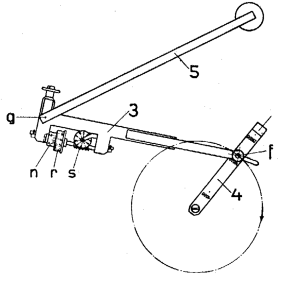This instrument, invented by Jacob Amsler (1823-1912) was used for computing the area enclosed by a closed curve.
The arm OB (first figure below) has a pivot point in O which is fixed to the paper. In our Siemens planimeter (top figure), O is a sharp point pressed into the paper by a weight.
Point B, which can describe a circle around O, rests in turn on a point of the arm AC. Point A is is moved by the user along the curve drawn on the paper. The other end of this arm rests on the paper with a steel wheel W, the axis of which is accurately parallel to AC. (In the Siemens planimeter, W is on the other side of A; also there is a supporting wheel perpendicular to W). The rotation of W can be measured accurately with the help of a division on its rim and a revolution counter. If AC is moved in its own direction, W slips over the paper without turning. However any movement component n normal to AC leads to a proportional rotation of W.If the original position of the arm is AB (next figure), and if it is moved to the nearby position A'B', then we have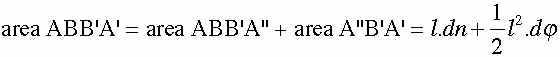if higher order terms are neglected (l is the length of AB).
If A makes a complete roundtrip over the closed curve K, then the surface covered by AB is equal to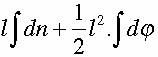This area is equal to the area enclosed by K because the area between the circle and K is covered twice in opposite directions. If O is outside K, then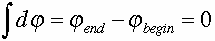Hence the area of K is proportional to the rotation of W, the constant of proportionality being determined by the dimensions of the planimeter.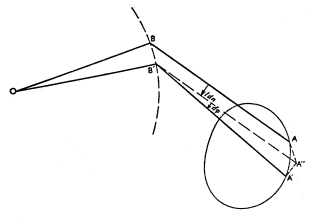In the Siemens planimeter drawing, part 4 is an accessory used for calibrating the instrument.

References: this explanation was adapted from the ENSIE encyclopedia (Amsterdam 1949). The top picture was taken from our Siemens Spezial-Planimeter manual (Berlin 1928).
Prof. Dr. Joachim Fischer (München) provided a number of corrections and additions.
A mathematical treatment of the planimeter, based on Green's Theorem, is given in this Wikipedia article
.